All submissions of the EM system will be redirected to Online Manuscript Submission System. Authors are requested to submit articles directly to Online Manuscript Submission System of respective journal.

# Original Article

, Volume: 12( 2)

## Batch and Column Studies for Phenol Removal from Aqueous Solutions using Laboratory prepared Low-Cost Activated Carbon as an Adsorbent

*Correspondence:
Arvind Kumar, Department of Chemical Engineering, National Institute of Technology, Rourkela-769008, Odisha, India, Tel: +91661246 2268; Fax: +91 661 247 2999; E-mail: [email protected]

Received: August 8, 2017; Accepted: September 8, 2017; Published: September 12, 2017

Citation: Lallan SY, Bijay KM, Arvind K. Batch and Column Studies for Phenol Removal from Aqueous Solutions using Laboratory prepared Low-Cost Activated Carbon as an Adsorbent. Chem Technol Ind J. 2017;12(2):114

### Abstract

The removal of phenol was studied using laboratory prepared activated carbons namely crab eye activated carbon (CEAC) using as a precursor crab eye (Abrus Precatorios) in a batch and column process. The effects of various parameters such as adsorbent dose, pH and temperature and contact time were investigated. Five kinetic models (pseudo-first-order, pseudo-second-order, intraparticle diffusion, Elovich and Bangham models) were tested to fit the experimental data. Among them Pseudo-second-order kinetic model was found to best fit representing the adsorption kinetic data. The Freundlich, Langmuir, Tempkin and Redlich-Peterson isotherm models were fitted to the experimental data of phenol adsorption. The different thermodynamic parameters such Gibbs free energy, enthalpy and entropy have also been estimated. The effects of important factors namely the flow rate (5 ml/min-15 ml/min), bed height (15 cm–25 cm) and influent concentration of phenol (50–15 mg/l) were studied in continuous fixed-bed adsorption for the removal of phenol from aqueous solution in crab eye activated carbon bed. Thomas, Adams-Bohart and Yoon-Nelson kinetics models were tested to experimental data for the prediction of the breakthrough point and to determine the characteristic parameters of the column.

### Keywords

Adsorption; phenol; Crab eye activated carbon; Kinetics; Isotherm; Column study

### Introduction

Phenol is frequently encountered in wastewater from various sources . Phenol is considered as a priority pollutants due to its toxicity to organism . Table 1 shows the concentration of phenol in various industrial effluents. The ingestion of 1 g of phenol may cause fatality to human . The presence of phenol in wastewater causes adverse impacts on environmental health . Therefore, several environmental regulating agencies of the world have set maximum permissible limit (Table 2) for phenol discharge into various water bodies [5-13]. Several conventional chemical, physical and biological methods have been established for the treatment of phenolic wastewater . Adsorption of environmental pollutants from wastewaters onto activated carbon is still considered to be the best option due to several reasons [12,14-15]. However, use of activated carbon as an adsorbent presents several disadvantages [1,16-20]. During past decade, several low-cost renewable materials have been used as an activated carbon after altering their surface characteristic for use in the treatment of wastewater. These low cost adsorbents have been made from lignocellulosic materials such as agricultural waste materials, industrial waste and plant residues .

Industries Concentrations (mg/l)
Oil refineries 6-500
Coking operations 28-3900
Coal processing 9-6800
Petrochemicals 2.8-1220
pharmaceutical, plastics, wood products, paint and pulp and paper industries 0.1-1600

Table 1: Phenol concentrations in industrial effluents.

Agency Type of water Maximum permissible limit
USEPA Surface water <0.1 ppb
BIS Drinking water 1.0 ppb
WHO Drinking water 1.0 ppb
MoEF Industrial effluent 1.0 ppm
Italy Potable and mineral waters 0.5 ppb
Surface waters 0.5 ppm
Sewerage system 1 ppm

Table 2: Maximum permissible limits of phenol in water.

This paper first time reports usage of crab eye activated carbon (CEAC) prepared from crab eye seeds as a precursor for the adsorption of phenol from aqueous solutions using batch and fixed bed operations. Several experimental factors which affect the removal of phenol such as adsorbate-adsorbent contact time, initial solution pH, adsorbent dosage, adsorbate concentration and adsorption temperature were optimized. The effects of parameters as flow rate, initial phenol concentration and bed height on the breakthrough curves were investigated. The maximum sorption capacity of CEAC onto phenol was also determined using Thomas model.

### Materials and Methods

Preparation of activated carbon

The high carbon content, cost and availability of raw materials are the some of the important criteria for their selection . In the present work, new adsorbent has been explored that has good adsorption capacity as compare to the commercial grade activated carbon. The raw material (Figure 1a) for the development of activated carbon was obtained from the campus of National Institute of Technology (NIT), Rourkela.

The tubular reactor (rectangular horizontal furnace having crossectional area 3 × 3 in square and length 30) was used for the production of activated carbon. The precursor sample was pyrolyzed at 750 K for 5 h under the N2 atmosphere. The sufficient amount of carbonized material was treated with saturated solution of NaOH for activation. In some cases the alkali treatment has increased adsorption capacity [22-24]. However, various researchers have used others chemical agent also for activation of biomass such as formaldehyde , sodium hydroxide [23,24, 26-28], washing with cold or hot water [24,26,28], with sulfuric acid , phosphorus (V) oxychloride , ac tivation of corn cobs using potassium hydroxide  etc for the surface modification of adsorbent. The sample was then dried in an oven at temperature of 115°C for 8 h. This mixture was then heated to 450°C–650°C for 2–3 h in a nitrogen atmosphere in the horizontal furnace with a heating rate of 5°C/min. The material was taken out from the furnace and allowed to cool to ambient conditions. After chemical activation, the final products were washed with double distillated water (DDW) to remove chemicals from the final products and to reach the pH=6-7 of the residual solution. All the water soluble impurities were removed by washing with DDW [32-33]. Immediately after washing, the sample was dried in the oven at the temperature of 110°C for 120 min. Activated carbon thus prepared was named as crab eye activated carbon (CEAC) and was preserved for end use (Figure 1b). The physico-chemical characterizations (Table 3) of CEAC were done using standard methods [34-36].

Characteristics CEAC
Proximate analysis
Moisture (%) 1.91
Volatile matter (%) 33.33
Ash (%) 8.66
Fixed Carbon (%) 58.01
Bulk density (kg/m3) 0.7701
Dry density 0.7610
Porosity 0.2286
Specific gravity 0.9864
Void ratio 0.1660
Heating value (cal./g) 5929
Average particle size (μm) 478.73
Chemical analysis of ash
Insoluble (%) 65.17
SiO2 (%) 12.6
Fe2O3& Al2O3 (%) 3.38
CaO (%) 17.4
MgO (%) 0.96
Others 0.49
Carbon (%) 60.77
Hydrogen (%) 3.53
Nitrogen (%) 4.08
Sulfur (%) 1.20
EDX Analysis
Carbon 51.61
Nitrogen 23.84
Oxygen 21.15
Magnesium -
Phosphorus 2.15
Sulfur 0.01
Potassium -
Calcium -
Surface area of pores (m2/g)
(i) BET 265
BJH cumulative pore volume (cm3/g)
(i) Single point total 0.0388
(iii) BJH desorption 0.0352
Average pore diameter (?)
(i) BET 34.66
(iii) BJH desorption 58.34
Iodine No. 315
Methylene blue No. 150
Boehm Titration
Surface acidity (mmol/g) 6.53
Surface alkalinity (mmol/g) 3.00

Table 3: Physico-chemical characteristics of adsorbent.

Preparation of adsorbate stock solutions and analytical measurement

The stock solutions of phenol concentration 1000 mg/L were prepared by dissolving appropriate amount of phenol in distilled water. Desired concentrations were prepared from the respective stock solutions, making fresh dilutions for each adsorption study. The concentration of phenol was determined by measuring the absorbance of the aqueous solution at λmax=470 nm using a UV/vis spectrophotometer. The calibration plot of absorbance vs. concentration for phenol showed a linear variation up to a phenol concentration of 50 mg/l. Because the value of Co was kept between 25 and 150 mg/l, the samples were subsequently diluted with distilled water, whenever necessary, so that the phenol concentrations of the samples could be determined accurately using the linear portion of the calibration curve.

Point of zero charge and surface groups quantification

The pH at which the surface charge of adsorbent is zero, is called the point of zero charge (PZC). The surface is positively charged at pH<pHPZC and negatively charged at pH>pHPZC [37-39]. The PZC of CEAC was determined to be 6.25 as per the method presented . Surface groups (acidic and basic) present on the adsorbent surfaces (Table 3) was determined using Boehm titration .

In the batch adsorption study, the q=(%R x Co)/(100 × m) was used to determine the uptake capacity of phenol. Where, Co is the initial concentration of phenol, m is mass of adsorbent and %R is the percentage phenol removal. Phenol adsorption rate onto the adsorbent was determined by analyzing residual phenol after different contact intervals of 15 min to 360 min. The concentrations of adsorbent were varied between 0.2 to 7 g/l to determine the optimum dosages. To elucidate the effect of pH on phenol adsorption, the initial pH of phenol solution was varied in the range of 2–12 using 0.1 M HCl or 0.1 M NaOH. The effect of temperature on phenol adsorption on adsorbent was also studied in the range of 303K-333 K. For the fixed bed column study of phenol onto CEAC, the experiments were carried out in plexiglass column of 25 cm length having inside diameter (2 cm). Schematic diagram of experimental set up is shown in Figure 2. The column was provided with a feed port at the bottom centre of the column.

### Results and Discussion

Characterization of CEAC

The nitrogen adsorption-desorption isotherm and pore size distributions of the CEAC is shown in Figure 3 and 4, respectively. BJH method is the most popular method used for the evaluation of the mesoporous structure of the CEAC. Pore sizes are classified in accordance with the classification adopted by the International Union of Pure and Applied Chemistry (IUPAC) , that is, micro-pores (diameter (d)<20 Å), meso-pores (20 Å<d<500 Å) and macro-pores (d>500 Å). Micro-pores can be divided into ultra micro-pores (d<7 Å) and super micro-pores (7 Å<d<20 Å). Because of the larger sizes of liquid molecules, the adsorbent for liquid phase adsorbates should have predominantly mesoporous in the structure .

Pore size distributions of CEAC results are given in Table 3. The determination of specific surface areas (SSA) (m2/g) of CEAC was calculated using the BET equation as proposed in 1938 . The determination of SSA was done using N2 as adsorptive by estimating the volume of the adsorbed monolayer. As shown in Figure 5, the plot 1/[Q (po/p-1)] vs. (po/p) provide straight lines at low pressure range, usually 0.05<(P/Po)<0.25, depending on the sample. While the Langmuir specific surface area of the CEAC was estimated by plotting p/Q vs. pressure as shown in Figure 6. The BET surface area of CEAC was 265 m2/g.

To find out the optimum dosage of adsorbents, the adsorbent concentrations were varied from 0.2 to 7 g/l at fixed initial phenol concentration of 100 mg/l (Figure 7). It was observed that on increasing the adsorbent dosage the uptake capacity of phenol decreases, while the %R increases because m is inversely proportional to the uptake capacity. The point where these two curves cut together is regarded as optimum adsorbent dosage, which was found to be 0.4 for CEAC. Beyond this dosage, there is limited availability of adsorption sites and do not contribute much for further removal of phenol. Various authors have been reported similar finding for phenol adsorption onto activated carbon prepared from various materials [2,18,45].

Figure 7: Effect of adsorbents dosage on the removal of phenol CEAC (Co =100 mg/l, pHo=6, T =303 K, t =5 h).

Effect of pH

pH of the solution is key factor and play very important role for the removal of pollutants from waste stream. Adsorption of phenol by CEAC (0.4 g/L) was studied in the pH range of 2-12 (Figure 8). Phenol removal was maximum and constant for pH ≤ 8. Further, increase in pH (>8) lead to decrease in phenol removal. This decrease in phenol removal with increase in pH can be explained based on the point of charge. As explained in section 2.3, the surface of CEAC at higher pH became negatively charged and therefore repelled C6H5O- ions because of which the adsorption of phenol got reduced [37,39,49]. Thus, pH=8 was taken as an optimum pH for phenol-CEAC system.

Figure 8: Effect of initial pH on the equilibrium uptake of phenol(Co=100 mg/l, m=0.4 g/l for CEAC T=303 K, t=5h).

Effect of contact time and initial concentrations

To find out the optimum contact time for maximum adsorption of phenol onto adsorbent, experiments were done for varying time periods between 5 min and 360 min at optimum pH and dosage by fixing the phenol concentration in solution to 100 mg/l (Figure 9). The adsorption rate for phenol was initially very fast, such that adsorption of ~12-30 mg/g was observed within the first 15 min of phenol–adsorbent contact time. After that, adsorption rate slowed down due the competition among C6H5OH2+ to grab the remaining available adsorption sites. Adsorption equilibrium was achieved after 5 h resulting in more than ~28-43 mg/g removal of phenol, indicating the saturation of available adsorption sites. Hence, 2 h were taken as optimum contact time for phenol-CEAC system.

Figure 9: Effect of contact time and initial concentrations on the removal of phenol onto CEAC(Co=50-150 mg/l, m=0.4 g/l,pHo=8, T=303 K).

The batch experiments were conducted to elucidate the effect of Co on the adsorption of phenol onto CEAC. It was observed that the uptake of phenol increases with an increase in Co . The increase in adsorption uptake with an increase in Co is attributed to an increase in the driving force for adsorption and a decrease in resistance to the uptake of phenol by the adsorbent from the solution .

Several kinetic models (Table 4) have been used in the present study to investigate the adsorption process of phenol. The details of all these kinetic models are presented elsewhere [50-52]. Pseudo-first and second-order equations have been solved by using non-linear technique and ks and qe were obtained. Figure 9, shows a representative plot of qt vs. t (experimental and calculated) for adsorption of phenol onto CEAC for Co =50-150 mg/l at the optimum dosage, pH and temperature. The bestfit values of h , qe and kS along with the correlation coefficients for the pseudo-first-order and pseudo-second-order models are given in Table 4. The qe,exp and qe,cal values (Table 4) from the pseudo-second-order kinetic model are very close to each other. The calculated correlation coefficients are equal to unity for pseudo-second-order kinetics than that for the pseudo first-order kinetic model. Therefore, the sorption of phenol can be approximated more appropriately by pseudo-second-order kinetic model than the other kinetic models for the adsorption.

Pseudo-first-order:qe=equilibrium uptake
Concentration (mg/l) 50 mg/l 75 mg/l 100 mg/l 150 mg/l
qe,exp(mg/g) 12.16 21.34 28.87 32.96
qe,calc(mg/g) 10.91 21.10 25.83 32.64
kf (min-1) 0.0185 0.0196 0.0186 0.0170
R2 0.9751 0.9965 0.9719 0.9679
MPSD 15.38 7.77 16.02 27.87
Pseudo-second-order:ks=2nd order rate constant
qe,calc (mg/g) 14.20 25.51 33.90 35.64
h (mg/g. min) 0.2357 0.4769 0.5574 0.9968
ks(g/mg. min) 0.0012 0.0007 0.0005 0.0008
R2 0.9902 0.9812 0.9888 0.9676
MPSD 11.46 14.59 12.18 20.03
Intra particle diffusion:kint=intra-particle diffusion rate constant (mg g-1 min0.5)
C=boundary layer effect
kint1(mg/g. min1/2) 0.7545 0.9604 1.2956 7.3305
C1(mg/g) 24.49 46.44 63.61 41.90
R2 0.6246 0.739 0.6909 0.9622
kint2 (mg/g. min1/2) - - - 0.2394
C2 (mg/g) - - - 104.86
R2 - - - 0.8237
Elovich:a=initial rate (mg/g.min)
b=parameter (g/mg) related to the extent of surface coverage and activation energy
b (g/mg) 0.216 0.193 0.141 0.082
a (mg/g. min) 83.91 3283 4480 400
R2 0.8218 0.8908 0.8543 0.9006
Bangham:(<1) and  are constants
α 0.30 0.81 0.20 0.25
ko 11.075 13.50 20.72 13.59
R2 0.911 0.965 0.967 0.965

Table 4: Kinetics parameters constants for pseudo-first-order, pseudo-second-order, intraparticle diffusion, Elovich and Bangham models.

If the Weber-Morris plot of qt vs. t0.5 does not satisfies the linear relationship with the experimental data, then the sorption process is not found be controlled by intra-particle diffusion. However, if the data exhibit multi-linear plots, then two or more steps influence the sorption process. The constants of Elovich equation are determined from the plot of qt vs. ln t. All the constants of Elovich are given in Table 4.

Since, the plot ofplot for phenol adsorption onto CEAC for Co =50-150 mg/l at 303 K and at optimum pH, did not yield linear curves and the values of R2≠1. This shows that the diffusion of phenol into the pores of adsorbents is not the only rate-controlling .

Adsorption diffusion and mass transfer study

In order to estimate whether the sorption process is rate limiting step mean either pore diffusion or surface diffusion or external mass transfer means boundary layer diffusion. The kinetic data as obtained by conducting batch experiments have been tested by the model given by Boyd :(1)

and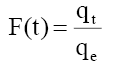(2)

Where qt and qe are the amount of phenol adsorb at any time t and equilibrium (mg/g). F(t) is fractional amount of phenol adsorb and Bt is a function of F (t).

Substituting eqn. 1 in eqn. 2, it simplifies to:(3)

The fitting of data plot (linear lines) Bt vs. t (min) for all phenol initial concentrations did not pass through the origin (Figure 10) indicating that the adsorption of phenol onto adsorbents was mainly due to external mass transport where particle diffusion (exterior surface of the adsorbent) was the rate limiting step .

Figure 10: Boyd plot for the removal of phenol by CEAC(Co=50-150 mg/l, t=5h; m=0.4 g/l,pHo=8).

The Bt values were also used to calculate the effective diffusivity, De (cm2/s) using the relation :(4)

Where, r represents the particle radius estimated by sieve analysis by assuming as spherical particles. The value of average effective diffusion coefficient De =5.84 x 10-13 m2/s was determined for Co =50-150 mg/l using eqn. (3).

A mass transfer phenomenon was studied with the help of following equation,(5)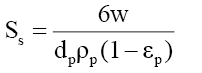(6)

Where, Co and Ct are the initial phenol concentration (mg/L) and concentration at time of t of the adsorbate respectively; m (g/L) is the mass of adsorbent. K (L/g) is the Langmuir constant, K1 (cm/s) is the mass transfer coefficient and Ss (L/cm) is the outer surface of the adsorbent per unit volume. According to above equation, surface mass transfer is controlling when tends to zero. A plot of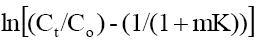vs. t results in a straight line (Figure 11). The value of K1 =1.02×10-7,0.483×10-7 and 0.316×10-7 cm/s were obtained at temperature 303, 313 and 323 K, respectively for Co =100 mg/l from slope and intercept of the straight line indicating that the transfer of phenol from bulk of the liquid to adsorbent surface is very rapid and the adsorbent is suitable for removal of phenol from aqueous solution. Similar finding related to determination of mass transfer coefficient has been reported earlier .

Figure 11: Mass transfer plot for adsorption of phenol onto CEAC system (Co=100 mg/I, t=5h; w=0.4 g/l,pHo=8).

The most appropriate equilibrium isotherm equation needs to be established to optimize the design of an adsorption system for the removal of adsorbates, which represents the experimental equilibrium sorption data. Several isotherm equations are available. The Freundlich and Langmuir isotherm equations have been used extensively to represent the equilibrium adsorption data obtained from activated carbon–organic contaminants systems, despite the fact that these equations have serious limitations on their usage [58-59]. Temkin isotherm equation contains a factor that explicitly takes into account the interactions between adsorbing species and the adsorbate. This isotherm assumes that (i) the heat of adsorption of all the molecules in the layer decreases linearly with coverage due to adsorbate–adsorbate interactions and (ii) adsorption is characterized by a uniform distribution of binding energies, up to some maximum binding energy . The Redlich–Peterson (R–P), a three-parameter-equation is often used for sorption data on heterogeneous surfaces and can be reduces to Freundlich equation at high concentrations and to Henry’s equation at very low concentrations .

Langmuir, Freundlich, Tempkin and Redlich-Peterson adsorption isotherms were tested for their fitness (Figure 12) over temperature range of 303 K-333 K. Calculated constants of all the isotherms and their corresponding non-linear regression coefficients at various temperatures are presented in Table 5. KF is the adsorption capacity at unit concentration and 1/n is the adsorption intensity. 1/n value indicates the type of isotherm to be irreversible (1/n=0), favorable (0<1/n<1) and unfavorable (1/n>1). Higher the value of 1/n, the higher will be the affinity between the adsorbate and the adsorbent and the heterogeneity of the adsorbent sites. The 1/n value indicates the relative distribution of energy sites and depends on the nature and strength of the adsorption process. For example, 1/n=0.57 refers to the fact that 57% of the active adsorption sites have same energy level . It is found from Table 5 that the CEAC is more heterogeneous in nature. Since 1/n<1, phenol is favorably adsorbed CEAC at all temperatures.

Figure 12: Equilibrium isotherm plot for the removal of phenol onto CEAC (Co=50-150 mg/I, t=2h; m=0.4 g/l,pHo=8, T=303K).

Freundlich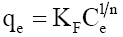Temperature (K) KF[(mg/g)/(mg/l)1/n] 1/n R2
303K 7.6577 0.5900 0.9741
313K 8.7655 0.5276 0.9876
323K 6.6652 0.5709 0.9846
333K 5.8573 0.5747 0.9980
LangmuirTemperature (K) qm(mg/g) KL(l/mg) R2
303K 169.3391 0.0175 0.9805
313K 141.9685 0.0201 0.9974
323K 143.4776 0.0161 0.9902
333K 139.3689 0.0138 0.9979
TemkinTemperature (K)  KT(l/mg) BT R2
303K 0.1370 41.4906 0.9891
313K 0.1562 34.7158 0.9975
323K 0.1271 34.9357 0.9929
333K 0.1153 32.8701 0.9971
Redlich-PetersonTemperature (K) KR(l/g) aR(l/mg) β R2
303K 1.9833 0.0000 4.3023 0.9451
313K 1.900 0.0000 3.3796 0.9471
323K 14.184 1.6551 0.4694 0.9751
333K 121.43 20.2302 0.4292 0.9964

Table 5: Isotherm parameters for the removal of phenol onto CEAC at different temperatures ( Co =50-150 mg/l; t =2 h; m=0.4 g/l; pHo 8).

The qm is the monolayer saturation at equilibrium, whereas KL corresponds to the concentration at which the amount of phenol bound to the adsorbent is equal to qm /2. This indicates the affinity of the phenol to bind with the CEAC. A high KL value indicates a higher affinity. The data in Table 6 also indicate that the values of qm and KL decreased with an increase in temperature confirming the exothermic nature of the overall sorption process. From Table 5, it can be seen that the value of regression coefficient is highest for Tempkin isotherm at all the temperatures indicating the fitness of Tempkin isotherm.

qe (mg/g) ?Hst, a (kJ/kg)
15 -15.70
20 -15.71
30 -15.73
40 -15.75
50 -15.76

Table 6: Isosteric enthalpy of phenol onto CEAC.

Effect of temperature

The effect of temperature on adsorption of phenol onto CEAC was investigated at 303 K, 313 K and 323 K, as shown in Figure 13. It can be seen that the adsorption capacity of CEAC decreases as the temperature increases. It can, therefore, be inferred that the adsorption is exothermic in nature.

Figure 13: Equilibrium adsorption isotherms at different temperatures for adsorption of phenol onto CEAC (Co=50-150 mg/I, m=0.4 g/l,pHo=8, t=2h).

Estimation of thermodynamic parameters

The thermodynamic parameters such as free energy of adsorption, enthalpyand entropychanges were estimated for the phenol adsorption onto CEAC by using equations presented in earlier publications [63-64]: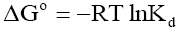(7)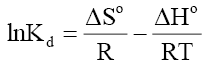(8)

Where, Kd is known as the distribution coefficient of the adsorbate, is equal to (qe / Ce ) . R is the gas constant (8.314J/mol.K) and T is the temperature in Kelvin. The plot of lnKd vs. 1/T is linear with the slope and the intercept giving values of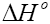and. These values were used to estimate.The Van’t Hoff plots for the adsorption of phenol onto CEAC are shown in Figure 14. The estimated values forwere found to be negative at the various temperature for various concentrations indicating that spontaneous physical adsorption [64-65]. The negative value of change in enthalpyconfirms that the adsorption of phenol to adsorbents is an exothermic process .

Figure 14: Van’t Hoff plot for the adsorption of phenol onto CEAC (Co =50-150 mg/l; t =2 h; m=0.4 g/l;pHo 8).

These thermodynamic parameters are given in Table 7. The heat of adsorption valuesbetween 0 and -20 kJ/mol are frequently assumed as to indicate physical nature of adsorption. Value forwas found to be −9.19 to 13.65 kJ/mol for phenol adsorption onto CEAC . It is obvious from thevalue that physio-sorption is mainly taking part in the adsorption process.

Co(mg/l) ΔHo(kJ/mol)  ΔSo(kJ/mol.K) ΔGo (kJ/mol K)
303K 313K 323K 333K
50 -9.88 31.25 -19.44 -19.95 -20.12 -20.42
75 -13.28 19.55 -19.04 -19.12 -19.49 -19.50
100 -13.65 17.45 -19.04 -19.12 -19.49 -19.50
125 -11.71 22.15 -18.56 -18.69 -19.00 -19.20
150 -9.19 28.35 -17.94 -18.18 -18.47 -18.79

Table 7: Thermodynamic parameters for phenol adsorption onto CEAC ( Co =50-150 mg/l; t =2 h; m=0.4 g/l; pHo 8).

Apparent isosteric heat of adsorptionat constant surface coverage (by considering the range of qe values obtained in each experiment) is calculated using Clausius-Clapeyron equation .(9)

or(10)

For this purpose, the equilibrium concentration ( C e ) at a constant equilibrium amount of adsorbed solute, qe , is obtained from the adsorption isotherm data at different temperatures.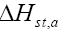is calculated from the slope of the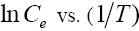plot for different qe of phenol onto CEAC. The isosters corresponding to different equilibrium adsorption uptake of phenol by CEAC is shown through Figure 15. The results shown in Table 7, suggest that eqn. (9) represents the experimental data very well.

The variation offor the adsorption of phenol with the surface loading is presented in Figure 16. Theis increasing with increasing surface loading indicating that CEAC has homogeneous surface. The dependence of heat of adsorption with surface coverage is usually observed to display the adsorbent-adsorbate interaction. The negative values of isosteric heat of adsorption for the adsorbate-adsorbent system shows that the sorption of phenol is an exothermic process .

Column study

The effectiveness of adsorption process is measured based on maximum adsorption capacity of adsorbate onto adsorbent. Batch study of phenol removal onto laboratory prepared low cost CEAC demonstrated the highest phenol uptake onto (~170 mg/g) onto CEAC. In the column study, the applicability of CEAC as an adsorbent in a packed column under continuous-flow conditions was evaluated for the phenol removal from industrial point of view due to several advantages such as high yield operations and relatively easy scaling up from a laboratory process etc. [69-70]. The effects of parameters such as flow rate, packed bed height and initial sorbate concentration were studied. Although a number of researchers have recently reported on column behavior with respect to the removal of several adsorbates from aqueous solutions, only a few articles have reported on the dynamics of phenol adsorption on packed beds [71-76].

The most important criterion in the design of a column adsorber is the prediction of the column breakthrough, which determines the bed length and the operating lifespan and regeneration time of the bed [77-78]. Factors that affect the column breakthrough include both operating variables and characteristics of the sorbate and the sorbent. Thomas, Adams-Bohart and Yoon-Nelson kinetics models have been used for the prediction of breakthrough times. The details along with limitations of these models are presented elsewhere .

Effect of various parameters on breakthrough curves: The effect of flow rate (5 ml/min, 10 ml/min and 15 ml/min) for the phenol adsorption onto the CEAC was studied using an inlet phenol concentration of 100 mg/l and bed height of 150 mm is shown in Figure 17a. The phenol uptake is observed fast in the initial stages and rate become slower thereafter and finally reached to saturation. As flow rate increased, the breakthrough curves become steeper and reached the breakthrough quickly. This is because of the residence time of the adsorbate in the column, which is long enough for adsorption equilibrium to be reached at high flow rate. This means that the contact time between the phenol and CEAC is minimized. Increasing the flow rate gave rise to a shorter time for saturation . The adsorption capacity of the CEAC was exhausted after 4-5 h of column operation. The breakthrough time for CEAC was found to be ~1.5 h for phenol.

Figure 17: Effect of (a) flow rate on breakthrough curve for phenol adsorption on CEAC (Inlet phenol concentration=100 mg/l; Bed height=150 mm), (b) bed height on breakthrough curve for phenol adsorption on CEAC (Bed Inlet phenol concentration=100 mg/l; Flow rate=10 ml/min), (c) initial ion concentration on breakthrough curve for phenol adsorption on CEAC (Bed height=150 mm; Flow rate=10 ml/min).

Figure 17b shows the breakthrough curves for the adsorption of phenol onto CEAC at various bed heights (15 cm, 20 cm and 25 cm) using the inlet concentration of 100 mg/l and flow rate of 10 ml/min. The results indicate that the throughput volume of the aqueous solution increased with increase in bed height, due to the availability of more number of sorption sites [79-80]. The equilibrium sorption capacity decreased with increase in bed height. The adsorption capacity of the CEAC was exhausted after 4-5 h of column operation. The breakthrough time for CEAC was found to be ~30 min for phenol.

The effect of inlet phenol concentration on the breakthrough curves at bed height of 150 mm and flow rate of 10 ml/min is shown in Figure 17c. It is seen that as the initial ion concentration increased from 50 mg/l, 100 mg/l and 150 mg/l, the break point time decreased. On increasing the initial ion concentration, the breakthrough curves became steeper and breakthrough volume decreased because of the lower mass-transfer flux from the bulk solution to the particle surface due to the weaker driving force [80-82]. At higher concentration the availability of the phenol for the adsorption sites is more, which leads to higher uptake of phenol at higher concentration even though the breakthrough time is shorter than the breakthrough time of lower concentrations. The adsorption capacity of the CEAC was exhausted after 5 h of column operation. The breakthrough time for CEAC was found to be ~80 min for phenol.

Column kinetic study: Thomas, Adams-Bohart and Yoon-Nelson kinetics models were tested to experimental data to predict the breakthrough curves by linear regression and to determine the characteristic parameters of the column that are useful for process design. The linear form of these three models is presented as follows:

Thomas model:(11)

Yoon-Nelson model: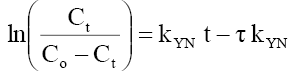(12)

Adams-Bohart model:(13)

Where Ct, Co =the effluent and inlet solute concentrations (mg/l), qe =the predicted adsorption capacity (mg/g), m=the total mass of the adsorbent (g), Q=volumetric flow rate (ml/min), V=the throughput volume (ml), kTh=Thomas rate constant (ml/min/mg), kYN the rate constant (l/min), τ is the time required for 50% adsorbate breakthrough (min), t is the breakthrough (sampling) time (min), kAB (l/min.mg) is rate constant of Adams-Bohart model, Z (cm) is the bed depth, No (mg/L) is maximum phenol adsorption capacity per unit volume of adsorbent column and uo (cm/min) is the linear velocity of influent solution.

The kinetic coefficient, kTh and adsorption capacity and Q for Thomas model were determined from plot of ln[(Co/Ce)-1] against t (Figure 18 a-c). The result of kTh , R2 and qo are given in Table 8. Thomas rate constant, kTh increases (0.0232-0.0335 ml/min/mg) as the flow rate increases, while its value decreases as initial phenol concentration (Co) and bed height increases. The maximum adsorption capacity, qt (mg/g) increased with increase in flow rate, initial ion concentration and bed height. The high values of regression coefficients were determined indicating that the kinetic data conformed well to Thomas model. It is interesting to note that so far none has reported such a high adsorption capacity (~890 mg/g) of phenol onto any low cost adsorbent in column study.

Figure 18: Linear plot of Thomas model with experimental data at (a) different flow rates (b) bed heights (c) different concentrations.

Kinetics Model Flow rate Bed height Initial concentration
5 ml/min 10 ml/min 15 ml/min 15 cm 20 cm 25 cm 50 mg/l 100 mg/l 150 mg/l
Thomas
kTh
(ml/min/mg)
0.0232 0.0289 0.0335 0.0293 0.0203 0.0224 0.0307 0.0179 0.0142
qT(mg/g) 342.82 538.91 665.83 618.77 712.62 815.44 413.28 701.55 889.5
R2 0.9792 0.995 0.9939 0.9841 0.9739 0.9722 0.9978 0.9982 0.9799
Yoon & Nelson
KYN(min-1) 0.0384 0.0478 0.55 0.0484 0.0335 0.0375 0.0267 0.0296 0.0352
τ (min) 165.69 130.33 106.52 149.83 172.73 197.44 190.08 169.7 145.29
qYN (mg/g) 2.0608 4.1217 6.1826 4.1217 3.2974 2.5364 2.171 4.1217 6.1707
R2 0.9792 0.995 0.9939 0.9841 0.9739 0.9722 0.9978 0.9982 0.9799
KAB
(ml/min.mg)
0.0178 0.0163 0.0181 0.0121 0.0097 0.0133 0.017 0.0083 0.0047
Madc(mg/L) 105.88 188.9161 240.4475 230.24 203.237 165.42 156.98 291.04 427.05
R2 0.9927 0.9579 0.9461 0.8292 0.8087 0.8206 0.9271 0.8874 0.8713

Table 8: Calculated columns Kinetics Parameters for phenol adsorption onto CEAC.

The kinetic parameters kYN (min-1), τ (min), qYN (mg/g) and regression coefficient R2 for Yoon-Nelson model were obtained by a plot of In Ce/(Co-Ce) vs. t gives a straight line with slope of kYN and intercept of –τ. kYN (Figure 19 a-c). The values of kYN , τ and adsorption capacity, qYN obtained are listed in Table 8. The results show that the rate constant, kYN increased with increased inlet phenol concentration and bed height but decreases with flow rate. Also, adsorption capacity, qYN increased with increase in flow rate and initial ion concentration but decreased with increase in bed height. The time required for 50% breakthrough, τ decreased with increase in flow rate, flow rate and initial ion concentration. High values of correlation coefficients indicate that Yoon and Nelson model fitted well to the experimental data.

Figure 19: Linear plot of Yoon-Nelson model with experimental data at (a) different flow rates, (b) different bed heights, (c) different concentrations.

Table 8 represents the linear regression results for Adams-Bohart model parameters. Linear plot of Adams-Bohart model in different heights, flow rates and concentrations is shown through Figure 20(a-c). The lower values of R2 as compare to the other models indicate that the Adams-Bohart model is not fitted well for the breakthrough curve.

Figure 20: Linear plot of Adams-Bohart model with experimental data at (a) different flow rates, (b) different bed heights, (c) different concentrations.

### Conclusion

Present study showed that CEAC was effective in removing phenol from aqueous solutions and thus can be used as low cost adsorbent. Removal of phenol was found to be strongly pH and temperature dependent. The experimental data were best fitted to pseudo-second-order kinetic model. The maximum sorption capacity of CEAC for phenol adsorption was found to be ~170 mg/g in batch experiments while it is remarkably very high ~890 mg/g in packed bed column study. The effect of temperature pronounced the negative effect on phenol adsorption. The adsorption experimental data fitted well to Temkin isotherm. The negative value of enthalpy of adsorption indicated that the adsorption of phenol onto crab eye activated carbon was exothermic in nature. In the column study of phenol removal onto CEAC, the breakthrough time was found to be significantly high as compare to other low cost adsorbents reported in literature. The low value of R2 as compare to the other models indicates that the Adams-Bohart model is not fitted well for the breakthrough curve.

### Funding Sources

The Authors are highly thankful to the Director of NIT Rourkela for providing financial assistance.

### Acknowledgement

The authors thank the Department of Chemical Engineering, National Institute of Technology and the head of the department for providing research facilities and constant encouragement.

### References

Select your language of interest to view the total content in your interested language

###### Citations : 214

Chemical Technology: An Indian Journal received 214 citations as per Google Scholar report

#### Indexed In

• CASS
• Open J Gate
• China National Knowledge Infrastructure (CNKI)
• CiteFactor
• Cosmos IF
• Directory of Research Journal Indexing (DRJI)
• Secret Search Engine Labs
• ICMJE

View More

#### For Librarians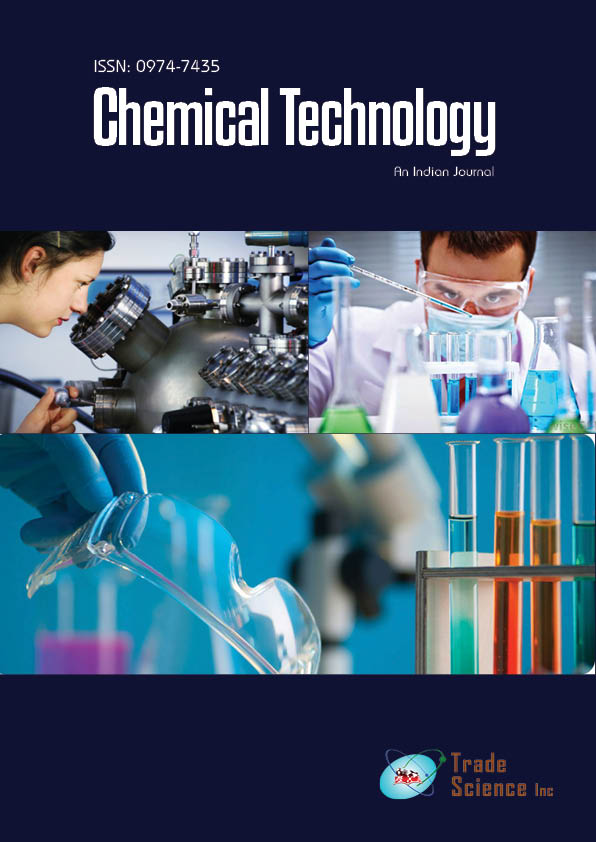#### Open Access Journals